Latest Banking jobs   »

# Reasoning Ability Quiz For SBI PO/Clerk Mains 2023- 3rd January

Directions (1-5): In each of the following questions two rows of numbers are given, the resultant number in such row is to be worked out separately based on the following rules and the question below the rows of numbers is to be answered. The operation of numbers progresses from left to right Rules.
(i) If an even number is followed by an odd number, then the numbers are to be added.
(ii) If an even number is followed by an even number, then the smaller number must be added to the half of the larger number.
(iii) If an odd number is followed by an odd number, then the smaller number must be subtracted from the larger number.
(iv) If an odd number is followed by an even number, then the larger number of the two is to be doubled.

Q1. If ‘m’ is the resultant of the first row, what will be the resultant of the second row?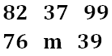(a) 97
(b) 95
(c) 98
(d) 92
(e) None of these

Q2. What is the sum of the resultants of the two rows, if ‘K’ is the resultant of the first row?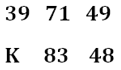(a) 110
(b) 105
(c) 107
(d) 102
(e) None of these

Q3. What is the difference of the resultant of both the rows?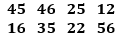(a) 112
(b) 127
(c) 139
(d) 122
(e) None of these

Q4. What is the sum of the resultant of both the rows?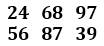(a) 226
(b) 227
(c) 259
(d) 240
(e) None of these

Q5. What is the multiplication of the resultant of both the rows?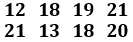(a) 640
(b) 760
(c) 820
(d) 920
(e) None of these

Directions (6-10): Study the following data carefully and answer the questions accordingly
In a coded language,
‘Every manual has rules’ is coded as ‘6&H 8#E 8@L 3%U’
‘Even rust harmful effects’ is coded as ‘1@U 10#C 1#V 10&F’
‘Mostly your_____(A)___ is coded as ‘1+O 8@I 3% T’
“Make ritual warm’ is coded as ‘1*A ___(B)___ 1%A’

Q6. What will be the code for the word ‘Honest Employee’?
(a) 3#E 5&Y
(b) 3&Y 5#E
(c) 3&E 5#Y
(d) 3*E 5%Y
(e) None of these

Q7. Which of the following code will come in the place of (B)?
(a) 3@U
(b) 3*U
(c) 3#T
(d) 3*U
(e) None of these

Q8. What will be the code for the word ‘Meritorious’?
(a) 8#I
(b) 14%U
(c) 14%O
(d) 8*U
(e) None of these

Q9. Which of the following word will come in the place of (A)?
(a) Roast
(b) Fairy
(c) Raise
(d) Retry
(e) None of these

Q10. What will be the code for the word ‘Miser’?
(a) 8%S
(b) 8*S
(c) 8%E
(d) 3%S
(e) None of these

Directions (11-15): In a certain code language, the following codes are used:
“Daily news selection post is coded as “@8d !2n \$4z #2d”
“Logical calculation ___(A)___ process” is coded as “@10z >4O !4q &6n”
“Conditional movement over strategy” is coded as “%6n >4u =4s &10n”
“Solve multiple instructions explained” is coded as “\$6t %8m >8w <4n”

Q11. Which of the following word will come in the place of (A)?
(a) Speed
(b) Method
(c) Mistake
(d) Solution
(e) None of these

Q12. What is the statement for the code “%6n \$4z”?
(a) News over
(b) Daily movement
(c) Strategy selection
(d) Conditional post
(e) None of these

Q13. What is the code for “Solve “?
(a) <4n
(b) @10z
(c) >4O
(d) !8m
(e) None of these

Q14. What is the code for “Logical”?
(a) @10z
(b) &6n
(c) >6w
(d) \$6t
(e) None of these

Q15. What is the code for “Calculation Explained”?
(a) @10z >8w
(b) &6n @10z
(c) >6w &10n
(d) \$6t >8w
(e) None of these

Solutions

S1. Ans. (a)
Sol. In row 1, according to the given step, resultant of row 1 is 20. So, m = 20.
In row 2, according to the given step, resultant of row 2 is 97.

S2. Ans. (c)
Sol. In row 1, according to the given step, resultant of row 1 is 81. So, K = 81
In row 2, according to the given step, resultant of row 2 is 26.
Hence, required sum = 81+ 26 = 107.

S3. Ans. (b)
Sol. In row 1, according to the given step, resultant of row 1 is 234.
In row 2, according to the given step, resultant of row 2 is 107.
Hence, required difference = 234 – 107 = 127.

S4. Ans. (c)
Sol. In row 1, according to the given step, resultant of row 1 is 155.
In row 2, according to the given step, resultant of row 2 is 104.
Hence, required sum = 155 + 104 = 259.

S5. Ans. (d)
Sol. In row 1, according to the given step, resultant of row 1 is 23.
In row 2, according to the given step, resultant of row 2 is 40.
Hence, required multiplication = 23 x 40 = 920.

Solution (6-10):
Sol. Every code has a number, alphabet and a special character.
The alphabet in each code is the 3rd alphabet from the right end of each word.
The number is decided such that if the word has even number of letters we subtract 3 from the number of letters, else if the number of letters is odd we add 3 to it.
Symbols: The words starting with ‘e’ are coded with #
R-@
H-&
M-%
Y – +
W – *

S6. Ans. (c)
S7. Ans. (a)
S8. Ans. (c)
S9. Ans. (c)
S10. Ans. (a)

Solution (11-15):
Sol.
The given code comprises a letter, symbol and a number.
The letter in the code is obtained after the second letter in the word from the left end is changed to the immediate previous letter of that letter in alphabetical series.
The number in the code is twice the number of vowels in the word.
The symbol in the code corresponds to the second letter from the right end.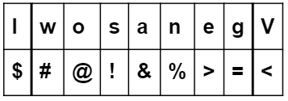S11. Ans. (a)
S12. Ans. (b)
S13. Ans. (a)
S14. Ans. (b)
S15. Ans. (a)## FAQs

#### Congratulations!